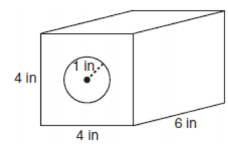mycollegehive
A solid metal prism has a rectangular base with sides of 4 i...
JMAP math geometry0A solid metal prism has a rectangular base with sides of 4 inches and 6 inches, and a height of 4 inches. A hole in the shape of a cylinder, with a radius of 1 inch, is drilled through the entire length of the rectangular prism.What is the approximate volume of the remaining solid, in cubic inches?

1) 19

2) 77

3) 93

4) 96

708 viewsShareFollowUniversity of Benin Nigeria
24 June 2020University of Lagos Nigeria
12 August 20200Since a cylindrical hole is drilled into the prism, the remaining volume, V will be the entire volume of the prism, V1 minus the volume of the drilled cylindrical shape, V2. That is,

V = V1 + V2

Where V1 and V2 are the volumes of the prism and cylinder respectively.

V1 = Length x Width x Height

V1 = 4 x 6 x 4

V1 = 96

V= πr2h

Where r is the radius and h is the height

V= π(1)2(6)

V= 19

Thus, V = 96 - 19

= 77

Ans 2Share

### Groups

How to insert math formulas/equations### Related Tags

JMAP

12 followers

143 questionsmath

36 followers

1262 questionsgeometry

13 followers

99 questions